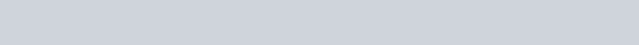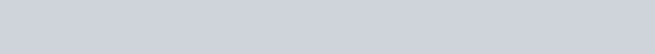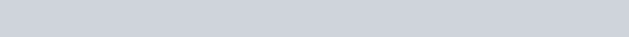# Skewness and Kurtosis in Statistics (shape of distributions)

Skewness and kurtosis are two important measure in statistics. Skewness refers the  lack of symmetry and kurtosis refers the peakedness of a distribution.

## Skewness

Literally, skewness means the ‘lack of symmetry’. We study skewness to have an idea about the shape of the curve which we can draw with the help of the given data. A distribution is said to be skewed if-
• Mean, median, mode fall at different points, i.e, Mean ≠ Median ≠ Mode.
• Quartiles are not equidistant from median.
• The curve drawn with the help of the given data is not symmetrical but stretched more to one side than the other.

The lack of symmetry in a distribution is always determined with reference to a normal distribution, which is always symmetrical. Any departure of a distribution from symmetry leads to an asymmetric distribution and in such cases, we call this distribution as skewed. The skewness may be either positive or negative. Absence of skewness makes the distribution symmetrical.

It is important to emphasize that skewness of a distribution cannot be determined simply by inspection. If we understand the differences between the mean, median and the mode, we should be able to suggest a direction of skew.We can define the skewness of a frequency distribution in three different shapes as following-

(1). Symmetrical distributions
This type of  distribution is known as normal distribution. We can obtain this distribution with height, weight, iq score and many other random variable from real life data. An important characteristics of such distribution is that the mean, median and mode have same value.

(2). Positively skewed distributions

In this distribution, the right tail is long which indicates the presence of extreme values at the positive end of the distribution. This pulls the mean to the right tail. this types of distribution is known as positively skewed distribution. This distributions occur with some real life variable such as family size, wages of the worker etc.

(3). Negatively skewed distributions
In this types of distribution, the mean is pulled in the negative direction. This occurs in some real life variable such as daily maximum temperature for a month in winter.

### Measures of skewness

We can simply measure the skewness using Pearson’s coefficient of skewness as below-

Skewness(p)= (Mean-Mode) / Standard Deviation

We can make following decisions from the Pearson’s coefficient of skewness as following-
• If mean > mode, the distribution is positively skewed.
• If mean < mode, the distribution is negatively skewed.
• If mean = mode, the distribution is not skewed or symmetrical.
In some case, mode cannot be uniquely defined, so we cannot apply the above formula. For alternative we use the following formula Pearson’s coefficient of skewness-

Skewness(p)= 3(Mean-Mode) / Standard Deviation

We can also measure the skewness using moments. The formula of skewness using moments is following-And Karl Pearson suggest skewness as,### Kurtosis

Kurtosis is a statistical measure that defines how heavily the tails of a distribution differ from the tails of a normal distribution. In other words, kurtosis identifies whether the tails of a given distribution contain extreme values.

### Measures of kurtosis

The measure of kurtosis is defined as the ratio of fourth central moment to the square of the second central moment.The measure is a pure number and is always positive.

Base on the value of kurtosis, we can classify a distribution as,
• If kurtosis>3, the distribution is leptokurtic.
• If kurtosis<3, the distribution is platykurtic.
• If kurtosis=3, the distribution is mesokurtic.

#### 1. Mesokurtic

Data that follows a mesokurtic distribution shows an excess kurtosis of zero or close to zero. This means that if the data follows a normal distribution, it follows a mesokurtic distribution.

#### 2. Leptokurtic

Leptokurtic indicates a positive excess kurtosis. The leptokurtic distribution shows heavy tails on either side, indicating large outliers. In finance, a leptokurtic distribution shows that the investment returns may be prone to extreme values on either side. Therefore, an investment whose returns follow a leptokurtic distribution is considered to be risky.

#### 3. Platykurtic

A platykurtic distribution shows a negative excess kurtosis. The kurtosis reveals a distribution with flat tails. The flat tails indicate the small outliers in a distribution. In the finance context, the platykurtic distribution of the investment returns is desirable for investors because there is a small probability that the investment would experience extreme returns.